# Reconstruction from Partial Tomography Measurements¶

Important: Please read the installation page for details about how to install the toolboxes. $\newcommand{\dotp}{\langle #1, #2 \rangle}$ $\newcommand{\enscond}{\lbrace #1, #2 \rbrace}$ $\newcommand{\pd}{ \frac{ \partial #1}{\partial #2} }$ $\newcommand{\umin}{\underset{#1}{\min}\;}$ $\newcommand{\umax}{\underset{#1}{\max}\;}$ $\newcommand{\umin}{\underset{#1}{\min}\;}$ $\newcommand{\uargmin}{\underset{#1}{argmin}\;}$ $\newcommand{\norm}{\|#1\|}$ $\newcommand{\abs}{\left|#1\right|}$ $\newcommand{\choice}{ \left\{ \begin{array}{l} #1 \end{array} \right. }$ $\newcommand{\pa}{\left(#1\right)}$ $\newcommand{\diag}{{diag}\left( #1 \right)}$ $\newcommand{\qandq}{\quad\text{and}\quad}$ $\newcommand{\qwhereq}{\quad\text{where}\quad}$ $\newcommand{\qifq}{ \quad \text{if} \quad }$ $\newcommand{\qarrq}{ \quad \Longrightarrow \quad }$ $\newcommand{\ZZ}{\mathbb{Z}}$ $\newcommand{\CC}{\mathbb{C}}$ $\newcommand{\RR}{\mathbb{R}}$ $\newcommand{\EE}{\mathbb{E}}$ $\newcommand{\Zz}{\mathcal{Z}}$ $\newcommand{\Ww}{\mathcal{W}}$ $\newcommand{\Vv}{\mathcal{V}}$ $\newcommand{\Nn}{\mathcal{N}}$ $\newcommand{\NN}{\mathcal{N}}$ $\newcommand{\Hh}{\mathcal{H}}$ $\newcommand{\Bb}{\mathcal{B}}$ $\newcommand{\Ee}{\mathcal{E}}$ $\newcommand{\Cc}{\mathcal{C}}$ $\newcommand{\Gg}{\mathcal{G}}$ $\newcommand{\Ss}{\mathcal{S}}$ $\newcommand{\Pp}{\mathcal{P}}$ $\newcommand{\Ff}{\mathcal{F}}$ $\newcommand{\Xx}{\mathcal{X}}$ $\newcommand{\Mm}{\mathcal{M}}$ $\newcommand{\Ii}{\mathcal{I}}$ $\newcommand{\Dd}{\mathcal{D}}$ $\newcommand{\Ll}{\mathcal{L}}$ $\newcommand{\Tt}{\mathcal{T}}$ $\newcommand{\si}{\sigma}$ $\newcommand{\al}{\alpha}$ $\newcommand{\la}{\lambda}$ $\newcommand{\ga}{\gamma}$ $\newcommand{\Ga}{\Gamma}$ $\newcommand{\La}{\Lambda}$ $\newcommand{\si}{\sigma}$ $\newcommand{\Si}{\Sigma}$ $\newcommand{\be}{\beta}$ $\newcommand{\de}{\delta}$ $\newcommand{\De}{\Delta}$ $\newcommand{\phi}{\varphi}$ $\newcommand{\th}{\theta}$ $\newcommand{\om}{\omega}$ $\newcommand{\Om}{\Omega}$

This numerical tour explores the reconstruction from tomographic measurement with TV regularization.

In :
addpath('toolbox_signal')


Width $n$ of the image.

In :
n = 128;


In :
name = 'phantom';


## Rotation over the Spacial Domain¶

Given an image $f$, its rotation is defined by rotating the pixels locations $$R_\th(f) = f(R_{-\th}(x)$$ where we have use the same notation $R_\th$ for the 2-D linear operator defined by the matrix $$R_\th = \begin{pmatrix} \cos(t) & -\sin(t) \\ \sin(t) & \cos(t) \end{pmatrix}$$

Computing rotation $R_\th(f)$ over a discrete grid requires interpolation.

In :
t = linspace(-1,1,n);
[Y,X] = meshgrid(t,t);
rotation = @(f,t)interp2(Y,X,f, sin(t)*X + cos(t)*Y, cos(t)*X - sin(t)*Y, 'cubic', 0);


## Rotation Over the Fourier Domain¶

A difficulty with spine-based rotation is that the corresponding operator is not exactly orthogonal.

In particular, one does not have $R_{-\th} = R_{\th}^{-1}$.

In :
clf;
imageplot(abs(rotation(rotation(f0,.2),-.2)-f0));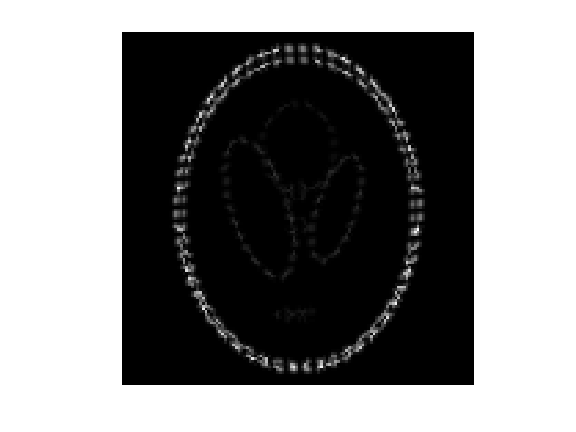To circunvent this problem, one can define the rotation using a Fourier-domain interpolation (a.k.a. Shannon). This is easily performed by decomposing a rotation as a series of shears which can themselves be implemented as diagonal operator over the Fourier domain.

X-aligned and Y-aligned shear matrices are defined as $$S_a^x = \begin{pmatrix} 1 & a \\ 0 & 1 \end{pmatrix} \qandq S_a^y = \begin{pmatrix} 1 & 0 \\ a & 1 \end{pmatrix}$$

One has the following decomposition of a rotation matrix as a product of shears matrices : $$R_\th = S_{-\tan(t/2)}^x \circ S_{\sin(t)}^y \circ S_{-\tan(t/2)}^y.$$

If we consider continuous functions defined over the plane $x \in \RR^2$ and define the Fourier transform along the X-direction as $$\hat f(\om,x_2) = \int f(x_1,x_2) e^{-i \om x_1} d x_2$$ one can compute the shear transformed function $$f_a(x) = f( S_{-a}(x) )$$ as a diagonal operator over the Fourier domain $$\hat f_a(\om,x_2) = e^{i \om x_2} \hat f(\om,x_2).$$

By analogy, we define the shear transform of a discrete image as a diagonal operator over the Fourier domain. Remark: to ensure that the transform is reald-valued, one should be careful about how to handle the highest frequency $n/2$, otherwise the transform would be complex-valued. Note also that this is an orthogonal transform (the eigenvalues have unit modulus).

In :
t = [0:n/2-1 0 -n/2+1:-1]';
[Y,X] = meshgrid(t,t);
shearx = @(f,a)real( ifft( fft(f) .* exp(-a*2i*pi*X.*Y/n) ) );
shearx = @(f,a)fftshift(shearx(fftshift(f),a));
sheary = @(f,a)shearx(f',a)';


Exercise 1

Display the effect of shearing $S_a^x$ for several values of shear $a$.

In :
exo1()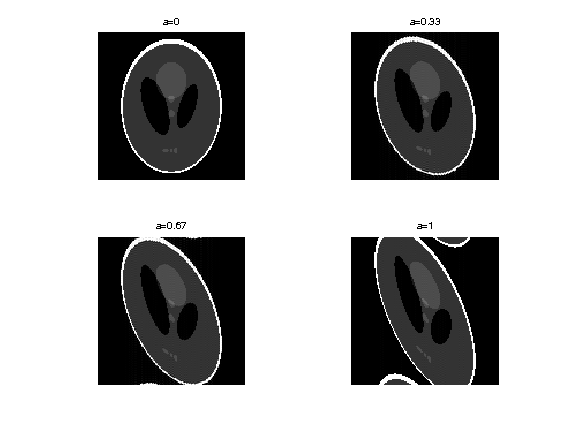In :
%% Insert your code here.


By analogy to the continuous setting, we define a discrete rotation by decomposing it using a series of shear.

In :
rotation = @(f,t)shearx( sheary( shearx(f,-tan(t/2)) ,sin(t)) ,-tan(t/2));


Exercise 2

Display the effect of rotations $R_\th$ for several values of angles $\th$.

In :
exo2()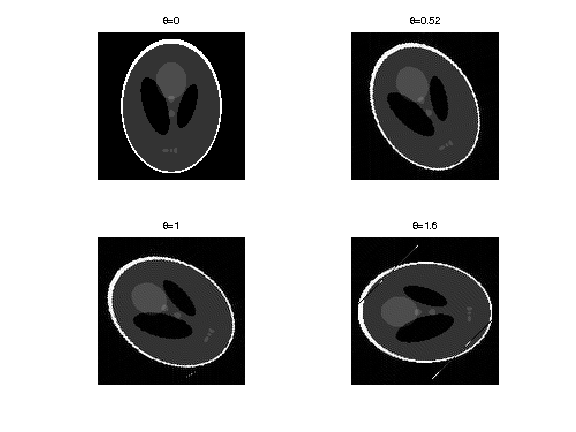In :
%% Insert your code here.


To avoid issues when using large angles, we replace a rotation of angle $\th$ by 4 rotations of angle $\th/4$. Note that this makes computation slower.

In :
rotation = @(f,t)rotation(rotation(f,t/2),t/2);
rotation = @(f,t)rotation(rotation(f,t/2),t/2);


Exercise 3

Display the effect of rotations $R_\th$ for several values of angles $\th$.

In :
exo3()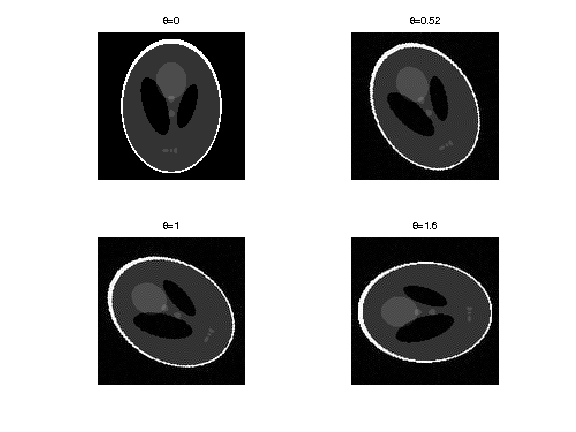In :
%% Insert your code here.


We check that this discrete rotation operator is exactly invertible, $R_{\th}^{-1} = R_{-\th}$.

In :
theta = .2;
e = norm( rotation(rotation(f0,theta),-theta)-f0 );
disp(['Error (should be 0): ' num2str(e, 2) '.']);

Error (should be 0): 1.3e-14.


We check that the inverse is also the adjoint operator, $R_{\th}^{-1} = R_{\th}^* = R_{-\th}$.

In :
theta = .1;
a = randn(n); b = randn(n);
dotp = @(a,b)sum(a(:).*b(:));
e = dotp(a,rotation(b,theta)) - dotp(b,rotation(a,-theta));
disp(['Error (should be 0): ' num2str(e, 2) '.']);

Error (should be 0): -1.3e-12.


The Radon transform is defined, for an angle $\th \in [-\pi/2,\pi/2)$ as an integration along all lines of angle $\th$. It can thus be defined equivalently by 1-D vertical integration of the image rotated by an angle $\th$. We use here a simple sum to replace the continuous integration: $$\Phi_\th(f)(x_1) = \sum_{x_2} R_\th(f)(x_1,x_2).$$

The radon transform is defined as the collection of projections for several angles, $\{ \th_i \}_{i=1}^{m}$, usually equi-spaced in $[-\pi/2,\pi/2)$. $$\Phi(f) = \{ \Phi_{\th_i}(f) \}_{i=1}^m \in \RR^{n \times m}.$$

For $y = \{y_{i}\}_{i=1}^m \in \RR^{n \times m}$, where $y_i \in \RR^n$, the adjoint of the Radon transform is $$\Phi^*(y) = \sum_i R_{-\th_i}(U(y_i)),$$ where $U : \RR^n \rightarrow \RR^{n \times n}$ replicates the vector along the lines, $U(v)(x_1,x_2)=v(x_1)$.

Number of angles for the partial tomography.

In :
m = 64;


List of angles.

In :
tlist = linspace(-pi/2,pi/2, m+1); tlist(end) = [];


Define the operators $\Phi$ and $\Phi^*$

In :
Phi  = @(f)perform_radon_transform(f, tlist, +1, rotation);
PhiS = @(R)perform_radon_transform(R, tlist, -1, rotation);


In :
clf;
imageplot(Phi(f0));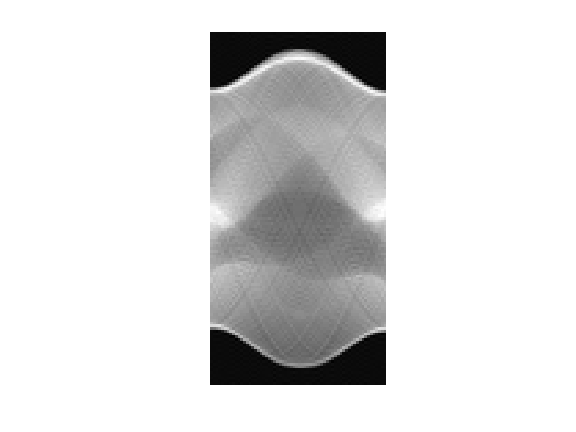Check that $\Phi^*$ is indeed the adjoint of $\Phi$.

In :
a = randn(n,m); b = randn(n);
e = dotp(a,Phi(b)) - dotp(PhiS(a),b);
disp(['Error (should be 0): ' num2str(e, 2) '.']);

Error (should be 0): 3.5e-12.


## Partial Tomography Pseudo-Inversion¶

We consider here a coarse sub-sampling of the Radon transform.

In :
m = 16;
tlist = linspace(-pi/2,pi/2, m+1); tlist(end) = [];
Phi  = @(f)perform_radon_transform(f, tlist, +1, rotation);
PhiS = @(R)perform_radon_transform(R, tlist, -1, rotation);


We use noiseless measurements $y=\Phi f_0$.

In :
y = Phi(f0);


Display the corresponing 1-D projections.

In :
clf;
plot(y); axis tight;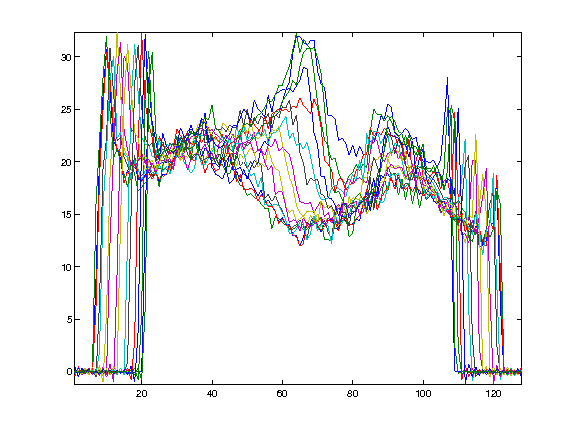The pseudo inverse reconstruction is defined as $$\Phi^+ y = \Phi^* ( \Phi\Phi^* )^{-1} y.$$ It is the minimum $L^2$ norm reconstruction $$\Phi^+ y = \uargmin{ f} \norm{y-\Phi f}^2.$$

Remark: When $m$ tends to infinity (continuous set of angles), the Radon transform is not sub-sampled and $\Phi \Phi^*$ is a filtering. For $y(r,\th)$ a continuous set of Radon coefficients, $$(\Phi\Phi^*) y(r,\th) = \int y(s,\th) h(r-s) d s \qwhereq \hat h(\om) = 1/\abs{\om}.$$ When $m$ is large enough, it is common to approximate $\Phi^+$ using $$\Phi^+ R = \Phi^*( g \star y )$$ where $g$ is a 1-D filter with $\hat g(\om) = \abs{\om}$ and $\star$ is the convolution along the first coordinate of the Radon coefficients.

This is not the case in our setting, so that $\Phi \Phi^*$ is actually difficult to invert, and it is thus computaitonaly difficult to compute the exact pseudo inverse $\Phi^+ = \Phi^* (\Phi \Phi^*)^{-1}$.

Exercise 4

Compute the pseudo inverse reconstruction using a conjugate gradient descent (function |cgs|).

In :
exo4()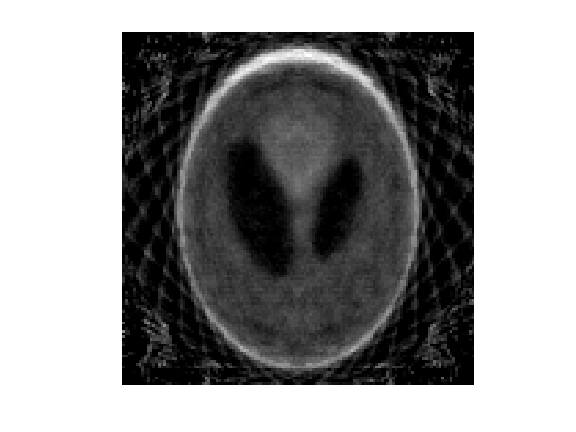In :
%% Insert your code here.


## Partial Tomography Total Variation Inversion¶

We consider the following reconstruction $$\umin{\Phi f = y} J(f) \qwhereq J(f)=\norm{\nabla f}_1$$ where the $\ell^1$ norm of a vector field $(u_i)_i$ is defined as $$J(f) = \sum_i \norm{u_i}.$$

WORK in PROGRESS

In :
return;


This problem is rewritten as $$\umin{f} F(K(f)) + G(f)$$ where $G=0$, $Kf = (\Phi f, \nabla f)$ and $F(u,v)=i_{\{y\}}(u) + \norm{v}_1.$

The primal-dual algorithm reads: $$g_{k+1} = \text{Prox}_{\sigma F^*}( g_k + \sigma K(\tilde f_k)$$ $$f_{k+1} = \text{Prox}_{\tau G}( f_k-\tau K^*(g_k) )$$ $$\tilde f_{k+1} = f_{k+1} + \theta (f_{k+1} - f_k)$$

One has $$\text{Prox}_{\la F}(u,v) = \pa{ y, \text{Prox}_{\la \norm{\cdot}_1}(v) }$$ where $$\text{Prox}_{\la \norm{\cdot}_1}(v)_k = \max\pa{0,1-\frac{\la}{\norm{v_k}}} v_k$$

In :
Amplitude = @(u)sqrt(sum(u.^2,3));
F1 = @(u)sum(sum(Amplitude(u)));
u = @(z)reshape(z(1:n*m),n,m);
v = @(z)reshape(z(n*m+1:end),n,n,2);

In :
ProxF1 = @(u,lambda)max(0,1-lambda./repmat(Amplitude(u), [1 1 2])).*u;
ProxF = @(z,lambda)[y(:); reshape(ProxF1(v(z),lambda),n*n*2,1) ];
ProxFS = @(y,sigma)y-sigma*ProxF(y/sigma,1/sigma);
ProxG = @(x,lambda)x;
KS = @(z)PhiS(u(z)) - div(v(z));


We set parameters for the algorithm. Note that in our case, $L=\norm{K}^2=n m$. One should has $L \sigma \tau < 1$.

In :
L = n*m;
sigma = 10;
tau = .9/(L*sigma);
theta = 1;

In :
f = fL2;


zeros(n);

In [ ]:
g = K(f)*0;
f1 = f;

In [ ]:
niter = 100;
E = []; C = []; F = [];
for i=1:niter
% update
fold = f;
g = ProxFS( g+sigma*K(f1), sigma);
f = ProxG(  f-tau*KS(g), tau);
f1 = f + theta * (f-fold);
% monitor the decay of the energy